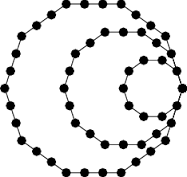GFG App
Open AppBrowser
Continue

# C program to find Decagonal Number

A decagonal number is a figurate number that extends the concept of triangular and square numbers to the decagon (a ten-sided polygon). The nth decagonal numbers counts the number of dots in a pattern of n nested decagons, all sharing a common corner, where the ith decagon in the pattern has sides made of i dots spaced one unit apart from each other. The n-th decagonal number is given by the formula D(n)=4n2-3n; The first few decagonal numbers are: 0, 1, 10, 27, 52, 85, 126, 175, 232, 297, 370, 451, 540, 637, 742, 855, 976, 1105, 1242……Examples:

```Input : n = 2
Output : 10

Input : n = 5
Output : 85

Input : n = 7
Output: 175```

## CPP

 `// C program to find nth decagonal number` `#include ` `#include `   `// Finding the nth  Decagonal Number` `int` `decagonalNum(``int` `n)` `{` `    ``return` `(4 * n * n - 3 * n);` `}`   `// Driver program to test above function` `int` `main()` `{` `    ``int` `n = 10;` `    ``printf``("Decagonal Number is = %d",` `           ``decagonalNum(n));`   `    ``return` `0;` `}`

Output:

`Decagonal Number is = 370`

Time complexity: O(1) as constant operations are done

Auxiliary space: O(1)